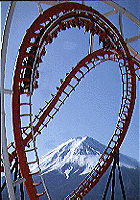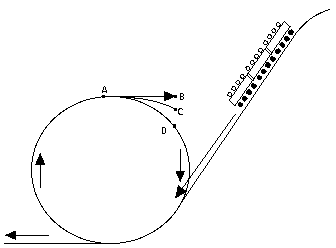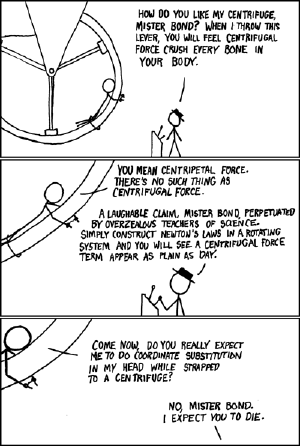# Disclaimer: The following material is being kept online for archival purposes.

## Although accurate at the time of publication, it is no longer being updated. The page may contain broken links or outdated information, and parts may not function in current web browsers.

 Note: In this lesson, as in the preceding one, all vector quantities will be underlined.

# (23a) Frames of Reference: The Centrifugal ForceIndex22.Reference Frames22a.Starlight Aberration22b. Relativity22c. Flight (1)22d. Flight (2)23. Inertial Forces23a. The Centrifugal Force23b. Loop-the-Loop24a.The Rotating Earth24b. Rotating Frames

The SunS-1. Sunlight & EarthS-1A. WeatherS-1B. Global Climate
 The schoolboy, rising early for his examination work, puzzled it out for himself .... "Centrifugal, centripetal," he said, with his chin on his fist. "Stop a planet in its flight, rob it of its centrifugal force, what then? Centripetal has it, and down it falls into the sun! And this--!" H.G.Wells, The Star   (click on the preceding to read the entire story)We now come back to motion in a circle.

## The Centrifugal Force

Motion in a circle is an accelerated motion. Therefore, if we study it in the rotating frame of reference, we can expect inertial forces to appear--like the ones discussed in the preceding section.

Suppose a person sits on a bus, moving in a straight line with constant speed v. As before, the forces involved are the passenger's weight F1 and the reaction force of the seat F2, and in the absence of acceleration, the two cancel:

F1+ F2 = 0

 Suddenly the bus makes a sharp turn, following part of a circle of radius r. If the passenger's body is to stay in the seat as before, an extra force must be added, to keep it from continuing in a straight line (its natural tendency). If ru is a unit vector (unit vectors are defined in the preceding section) directed outwards from the center of rotation, along the radius r, the force F2 exerted by the seat must increase to provide the centripetal force –(mv2/r)ru that makes the passenger follow the motion of the bus: F1+ F2 = – (mv2/r) ru   The bus and the seat now constrain the passenger's body to follow part of a circle, and to do this must pull it towards the center. To stay in place (that is, balance the above equation), F2 on it must be increased by an additional force in the direction of –ru, towards the center of the curve. How does this same event look in the frame of the bus? Adding (mv2/r)ru to both sides of the equation gives, in a similar way to what was done before F1+ F2 + (mv2/r) ru = 0 Equilibrium is attained and the passenger stays in place, if the equation above is satisfied. This can be viewed as the equilibrium between 3 forces-- F1, F2 and the centrifugal force (mv2/r)ru directed along ru, radially outwards. This can easily be generalized to any frame of reference moving around a circle:
 Equilibrium exists in the rotating frame if all forces balance, including an inertial "centrifugal" force (mv2/r)ru.

## Examples

When calculating the motions of the oceans and the atmosphere, it is much easier to use reference points on the rotating Earth and add a centrifugal force to all equations. That is one reason why the observed acceleration g due to gravity departs from the average value of 9.81: at the equator, the centrifugal force must be subtracted from the force of gravity, while at the pole no centrifugal force exists. Observations of g give values from 9.78 at the equators to 9.83 at the poles, but the centrifugal force is responsible for only part of that difference. The rest arises because the Earth is not a perfect sphere: the centrifugal force of its rotation causes its equator to bulge out, making the surface there more distant from the Earth´s center and thus weakening the gravitational pull.
(You may also note that the direction of the centrifugal force is away from the axis of rotation, and therefore it points away from the Earth´s center only at the equator. One therefore expects at all other points (except for the poles) a very slight difference between the vertical as defined by a plumb line and the direction to the center of the Earth.)Another example is the "loop the loop" feature found on some roller coasters in amusement parks. There the track descends on a long slope and then, at the bottom, turns in a complete circle (drawing) before leveling out again. At the point marked "A" the riders are briefly turned upside down, but no one ever falls out. How come? And (in the absence of friction), what is the minimum height h of the starting point S above A needed to ensure that the car passes safely through A? This is easily solved in the frame of the moving roller-coaster car. In that frame, the forces on the car at the top of the loop are The weight –mg ru (downwards, along –ru at this point.) The centrifugal force (mv2/r) ru, and The force F2 exerted by the rails.The car will just barely manage to get past point A if gravity and the centrifugal force are exactly equal, so that the rails need to exert no extra force: –mg ru + (mv2/r) ru = 0 The two vectors are both along ru (with signs giving the direction), so everyhing reduces to an equation between ordinary numbers: –mg + mv2/r = 0 Suppose now the car has come down from a height h above the highest point in the loop. It has lost potential energy mgh, and gained kinetic energy mv2/2 so from conservation of energy